• 昨天晚上参加阿里巴巴的实习面试，各种被虐。 伯努利分布最大似然估计
前言
昨天晚上参加阿里巴巴的实习面试，各种被虐。面试了将近90分钟，才做了3个题，加上项目的介绍。在机器学习方面，问到了一个伯努利分布的最大似然估计的推导，想到逻辑回归的推导就是利用最大似然估计，然后就套用了其推导过程。可能前面被说的有点迷糊了，导致最后也没有完整的推导出来，最失败的一次面试了。
对于阿里的暑期实习面试，其实问得内容还是都比较基础的。准备了很多关于特征工程、集成学习等内容，结果都没有考察到。反而被考的基础知识没有准备的比较清楚。这里对伯努利分布以及其最大似然估计做了一个详细的推导，其它的概率模型可以套用该模版。
祝看到此文章的小伙伴都能找到好的工作…

伯努利分布
伯努利分布，又名0-1分布，是一个离散概率分布。典型的示例是抛一个比较特殊的硬币，每次抛硬币只有两种结果，正面和负面。抛出硬币正面的概率为

p

p

，抛出负面的概率则为

1

−

p

1-p

。因此，对于随机变量

X

X

，则有：

f

(

X

=

1

)

=

p

f

(

X

=

0

)

=

1

−

p

\begin{aligned} f(X=1) &amp; = p \\ f(X=0) &amp; =1-p \end{aligned}

由于随机变量

X

X

只有 0 和 1 两个值，

X

X

的概率分布函数可写为：

(1)

f

(

X

)

=

p

x

(

1

−

p

)

1

−

x

0

&lt;

p

&lt;

1

f(X)=p^x(1-p)^{1-x}\qquad\text{$0&lt;p&lt;1$}\tag{1}

数学期望
在概率论和统计学中，数学期望（或均值）是试验中每次可能结果的概率乘以其结果的总和。它反映了随机变量平均取值的大小。
离散型
离散型随机变量

X

X

的数学期望为一切可能的取值

x

i

x_i

与对应的概率

p

(

x

i

)

p(x_i)

的乘积之和，即如果随机变量的取值为集合

{

x

1

,

x

2

,

⋯
&ThinSpace;

,

x

n

}

\lbrace x_1,x_2,\cdots, x_n \rbrace

，每个取值对应的概率为

{

p

(

x

1

)

,

p

(

x

2

)

,

⋯
&ThinSpace;

,

p

(

x

n

)

}

\lbrace p(x_1),p(x_2),\cdots, p(x_n) \rbrace

，则有：

(2)

E

(

X

)

=

∑

i

=

1

n

x

n

p

(

x

n

)

E(X) =\sum_{i=1}^{n}x_np(x_n) \tag{2}

因此，对于伯努利分布，其数学期望为：

E

(

X

)

=

1

⋅

p

+

0

⋅

(

1

−

p

)

=

p

E(X)=1\cdot p + 0\cdot (1-p)=p

对于随机变量

X

​

X​

，其方差和数学期望的公式满足：

(3)

V

a

r

(

X

)

=

E

(

(

X

−

E

(

X

)

)

2

)

=

E

(

x

2

)

−

[

E

(

x

)

]

2

Var(X)=E((X-E(X))^2)=E(x^2)-[E(x)]^2\tag{3}

一个随机变量的方差就是衡量随机变量和其数学期望之间的偏离程度。

公式推导如下：

V

a

r

(

X

)

=

E

(

(

X

−

E

(

X

)

)

2

)

=

E

(

X

2

−

2

X

⋅

E

(

X

)

+

[

E

(

X

)

]

2

)

=

E

(

X

2

)

−

2

⋅

E

(

X

)

⋅

E

(

X

)

+

[

E

(

X

)

]

2

=

E

(

X

2

)

−

[

E

(

X

)

]

2

\begin{aligned} Var(X)&amp;=E((X-E(X))^2)\\ &amp;=E(X^2-2X\cdot E(X)+[E(X)]^2)\\ &amp;=E(X^2)-2\cdot E(X) \cdot E(X) + [E(X)]^2\\ &amp;=E(X^2)-[E(X)]^2 \end{aligned}

对于伯努利分布，有

E

(

X

2

)

=

E

(

X

)

E(X^2)=E(X)

。因此，其方差为：

V

a

r

(

X

)

=

p

−

p

2

=

p

(

1

−

p

)

Var(X)=p-p^2=p(1-p)

最大似然估计
在统计学中，最大似然估计（MLE），也称为极大似然估计，是用来估计一个概率模型的参数的方法。其目的就是：利用已知的样本结果，反推最有可能导致这样结果的参数值。
由于样本集中的样本都是独立同分布，现以伯努利分布来推导其参数

p

p

最大似然估计。记已知的样本集为：

D

=

{

x

1

,

x

2

,

⋯
&ThinSpace;

,

x

n

}

D=\lbrace x_1,x_2,\cdots,x_n\rbrace

其似然函数为：

(4)

L

(

p

∣

x

1

,

⋯
&ThinSpace;

,

x

n

)

=

f

(

X

∣

p

)

=

f

(

x

1

,

x

2

,

⋯
&ThinSpace;

,

x

n

∣

p

)

=

∏

i

=

1

n

f

(

x

i

∣

p

)

=

∏

i

=

1

n

p

x

i

(

1

−

p

)

1

−

x

i

\begin{aligned} L(p|x_1,\cdots,x_n)&amp;=f(X|p)\\ &amp;=f(x_1,x_2,\cdots,x_n|p)\\ &amp;=\prod_{i=1}^n{f(x_i|p)}\\ &amp;=\prod_{i=1}^n{p^{x_i}(1-p)^{1-x_i}}\tag{4} \end{aligned}

由于有连乘运算，通常对似然函数取对数来计算，即对数似然函数。因此其对数似然函数为：

(5)

L

=

log

⁡

∏

i

=

1

n

f

(

x

i

∣

p

)

=

∑

i

=

1

n

log

⁡

f

(

x

i

∣

p

)

=

∑

i

=

1

n

[

x

i

log

⁡

p

+

(

1

−

x

i

)

log

⁡

(

1

−

p

)

]

\begin{aligned} L&amp;=\log \prod_{i=1}^nf(x_i|p)\\ &amp;=\sum_{i=1}^n{\log f(x_i|p)}\\ &amp;=\sum_{i=1}^n{[x_i\log p+(1-x_i)\log (1-p)]}\tag{5} \end{aligned}

等式

(

5

)

(5)

其实就是逻辑回归中使用到的交叉熵了。

p

^

=

arg

⁡

max

⁡

p

L

(

p

∣

X

)

=

arg

⁡

max

⁡

p

∑

i

=

1

n

[

x

i

log

⁡

p

+

(

1

−

x

i

)

log

⁡

(

1

−

p

)

]

\begin{aligned} \hat{p}&amp;=\arg \max_{p}L(p|X)\\ &amp;=\arg \max_p {\sum_{i=1}^n{[x_i\log p+(1-x_i)\log (1-p)]}} \end{aligned}

因此，最大似然估计其实就是求似然函数的极值点，将对数似然函数对参数

p

p

求导：

∂

L

∂

p

=

∑

i

=

1

n

[

x

i

p

+

1

−

x

i

p

−

1

]

=

∑

i

=

1

n

p

−

x

i

p

(

p

−

1

)

=

0

\begin{aligned} \frac{\partial L}{\partial p}&amp;=\sum_{i=1}^n{[\frac{x_i}{p}+\frac{1-x_i}{p-1}]}\\ &amp;=\sum_{i=1}^n{\frac{p-x_i}{p(p-1)}}=0 \end{aligned}

从而得到伯努利的最大似然估计为：

∑

i

=

1

n

(

p

−

x

i

)

=

0

&ThickSpace;

⟹
&ThickSpace;

p

=

1

n

∑

i

=

1

n

x

i

\begin{aligned} &amp;\sum_{i=1}^{n}{(p-x_i)}=0\\ \implies &amp;p=\frac{1}{n}\sum_{i=1}^n{x_i} \end{aligned}

总结
求概率模型的最大似然估计的一般步骤如下：
写出随机变量的概率分布函数；写出似然函数；对似然函数取对数，并进行化简整理；对参数进行求导，找到似然函数的极值点；解似然方程。
相信了解逻辑回归算法的小伙伴已经看出来了，对逻辑回归的推导其实质上也是最大似然估计算法。在逻辑回归中，其概率分布函数不再是

f

(

x

)

=

p

x

(

1

−

p

)

1

−

x

f(x)=p^x(1-p)^{1-x}

，而是：

(6)

P

(

y

∣

x

;

θ

)

=

(

h

θ

(

x

)

)

y

(

1

−

h

θ

(

x

)

)

1

−

y

P(y|x;\theta)=(h_{\theta}(x))^y(1-h_{\theta}(x))^{1-y}\tag{6}

其中：

(7)

h

θ

(

x

)

=

1

1

+

e

−

z

=

1

1

+

e

−

θ

T

x

h_{\theta}(x)=\frac{1}{1+e^{-z}}=\frac{1}{1+e^{-\theta^{T} x}}\tag{7}

详细过程参见：逻辑回归推导
展开全文机器学习
• ## 极大似然估计详解

万次阅读 多人点赞 2017-05-28 00:55:10
以前多次接触过极大似然估计，但一直都不太明白到底什么原理，最近在看贝叶斯分类，对极大似然估计有了新的认识，总结如下： 贝叶斯决策  首先来看贝叶斯分类，我们都知道经典的贝叶斯公式：  其中：p(w)：...
极大似然估计
以前多次接触过极大似然估计，但一直都不太明白到底什么原理，最近在看贝叶斯分类，对极大似然估计有了新的认识，总结如下：

贝叶斯决策
首先来看贝叶斯分类，我们都知道经典的贝叶斯公式：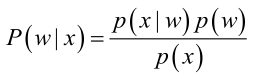其中：p(w)：为先验概率，表示每种类别分布的概率；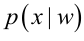：类条件概率，表示在某种类别前提下，某事发生的概率；而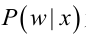为后验概率，表示某事发生了，并且它属于某一类别的概率，有了这个后验概率，我们就可以对样本进行分类。后验概率越大，说明某事物属于这个类别的可能性越大，我们越有理由把它归到这个类别下。
我们来看一个直观的例子：已知：在夏季，某公园男性穿凉鞋的概率为1/2，女性穿凉鞋的概率为2/3，并且该公园中男女比例通常为2:1，问题：若你在公园中随机遇到一个穿凉鞋的人，请问他的性别为男性或女性的概率分别为多少？
从问题看，就是上面讲的，某事发生了，它属于某一类别的概率是多少？即后验概率。
设：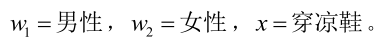由已知可得：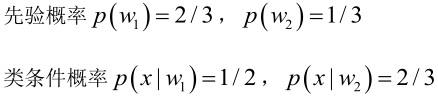男性和女性穿凉鞋相互独立，所以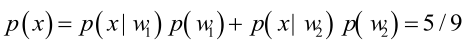（若只考虑分类问题，只需要比较后验概率的大小，的取值并不重要）。
由贝叶斯公式算出：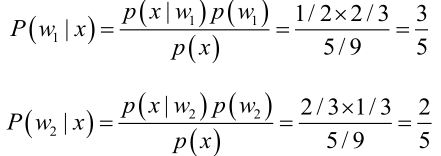问题引出
但是在实际问题中并不都是这样幸运的，我们能获得的数据可能只有有限数目的样本数据，而先验概率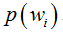和类条件概率(各类的总体分布)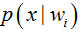都是未知的。根据仅有的样本数据进行分类时，一种可行的办法是我们需要先对先验概率和类条件概率进行估计，然后再套用贝叶斯分类器。
先验概率的估计较简单，1、每个样本所属的自然状态都是已知的（有监督学习）；2、依靠经验；3、用训练样本中各类出现的频率估计。
类条件概率的估计（非常难），原因包括：概率密度函数包含了一个随机变量的全部信息；样本数据可能不多；特征向量x的维度可能很大等等。总之要直接估计类条件概率的密度函数很难。解决的办法就是，把估计完全未知的概率密度转化为估计参数。这里就将概率密度估计问题转化为参数估计问题，极大似然估计就是一种参数估计方法。当然了，概率密度函数的选取很重要，模型正确，在样本区域无穷时，我们会得到较准确的估计值，如果模型都错了，那估计半天的参数，肯定也没啥意义了。

重要前提
上面说到，参数估计问题只是实际问题求解过程中的一种简化方法（由于直接估计类条件概率密度函数很困难）。所以能够使用极大似然估计方法的样本必须需要满足一些前提假设。
重要前提：训练样本的分布能代表样本的真实分布。每个样本集中的样本都是所谓独立同分布的随机变量 (iid条件)，且有充分的训练样本。

极大似然估计
极大似然估计的原理，用一张图片来说明，如下图所示：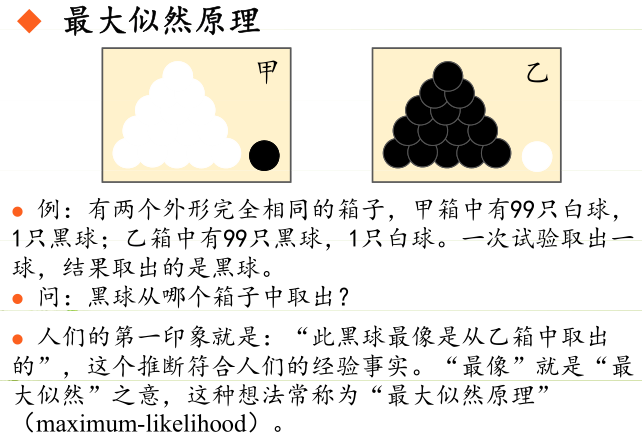总结起来，最大似然估计的目的就是：利用已知的样本结果，反推最有可能（最大概率）导致这样结果的参数值。
原理：极大似然估计是建立在极大似然原理的基础上的一个统计方法，是概率论在统计学中的应用。极大似然估计提供了一种给定观察数据来评估模型参数的方法，即：“模型已定，参数未知”。通过若干次试验，观察其结果，利用试验结果得到某个参数值能够使样本出现的概率为最大，则称为极大似然估计。
由于样本集中的样本都是独立同分布，可以只考虑一类样本集D，来估计参数向量θ。记已知的样本集为：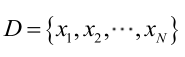似然函数（linkehood function）：联合概率密度函数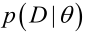称为相对于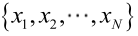的θ的似然函数。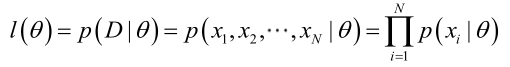如果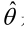是参数空间中能使似然函数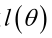最大的θ值，则应该是“最可能”的参数值，那么就是θ的极大似然估计量。它是样本集的函数，记作：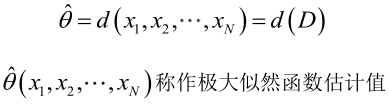求解极大似然函数
ML估计：求使得出现该组样本的概率最大的θ值。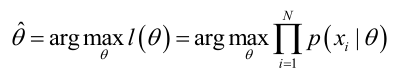实际中为了便于分析，定义了对数似然函数：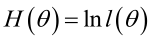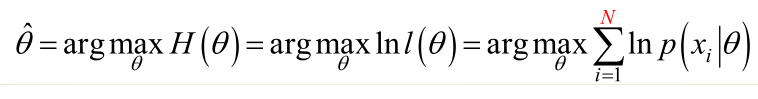1. 未知参数只有一个（θ为标量）
在似然函数满足连续、可微的正则条件下，极大似然估计量是下面微分方程的解：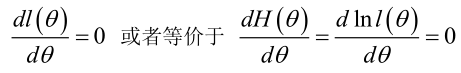2.未知参数有多个（θ为向量）
则θ可表示为具有S个分量的未知向量：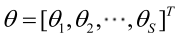记梯度算子：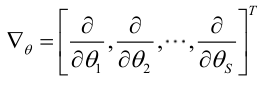若似然函数满足连续可导的条件，则最大似然估计量就是如下方程的解。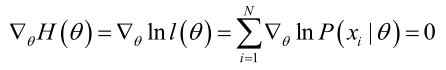方程的解只是一个估计值，只有在样本数趋于无限多的时候，它才会接近于真实值。

极大似然估计的例子
例1：设样本服从正态分布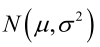，则似然函数为：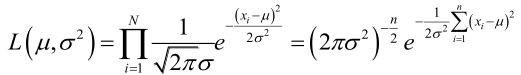它的对数：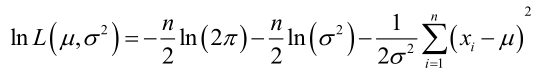求导，得方程组：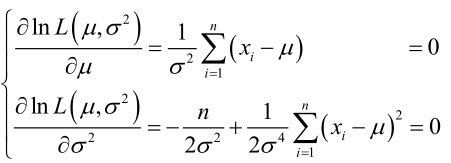联合解得：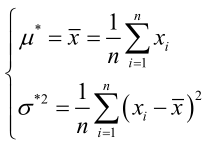似然方程有唯一解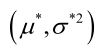：，而且它一定是最大值点，这是因为当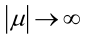或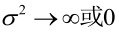时，非负函数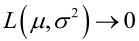。于是U和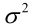的极大似然估计为。

例2：设样本服从均匀分布[a, b]。则X的概率密度函数：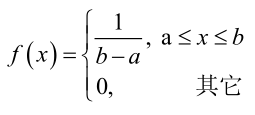对样本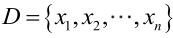：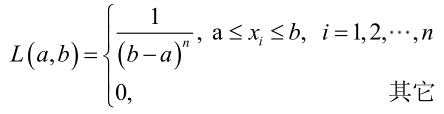很显然，L(a,b)作为a和b的二元函数是不连续的，这时不能用导数来求解。而必须从极大似然估计的定义出发，求L(a,b)的最大值，为使L(a,b)达到最大，b-a应该尽可能地小，但b又不能小于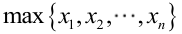，否则，L(a,b)=0。类似地a不能大过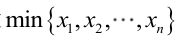，因此，a和b的极大似然估计：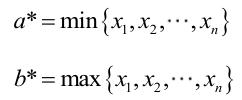总结
求最大似然估计量的一般步骤：
（1）写出似然函数；
（2）对似然函数取对数，并整理；
（3）求导数；
（4）解似然方程。
最大似然估计的特点：
1.比其他估计方法更加简单；
2.收敛性：无偏或者渐近无偏，当样本数目增加时，收敛性质会更好；
3.如果假设的类条件概率模型正确，则通常能获得较好的结果。但如果假设模型出现偏差，将导致非常差的估计结果。

正态分布ML估计的Matlab实例：点击打开链接
展开全文• 本文原始地址：最大似然估计（Maximum likelihood estimation, 简称MLE）和最大后验概率估计（Maximum a posteriori estimation, 简称MAP）是很常用的种参数估计方法，如果不理解这种方法的思路，很
声明：本文为原创文章，发表于nebulaf91的csdn博客。欢迎转载，但请务必保留本信息，注明文章出处。 本文作者: nebulaf91 本文原始地址：http://blog.csdn.net/u011508640/article/details/72815981

最大似然估计（Maximum likelihood estimation, 简称MLE）和最大后验概率估计（Maximum a posteriori estimation, 简称MAP）是很常用的两种参数估计方法，如果不理解这两种方法的思路，很容易弄混它们。下文将详细说明MLE和MAP的思路与区别。
但别急，我们先从概率和统计的区别讲起。
概率和统计是一个东西吗？
概率（probabilty）和统计（statistics）看似两个相近的概念，其实研究的问题刚好相反。
概率研究的问题是，已知一个模型和参数，怎么去预测这个模型产生的结果的特性（例如均值，方差，协方差等等）。 举个例子，我想研究怎么养猪（模型是猪），我选好了想养的品种、喂养方式、猪棚的设计等等（选择参数），我想知道我养出来的猪大概能有多肥，肉质怎么样（预测结果）。
统计研究的问题则相反。统计是，有一堆数据，要利用这堆数据去预测模型和参数。仍以猪为例。现在我买到了一堆肉，通过观察和判断，我确定这是猪肉（这就确定了模型。在实际研究中，也是通过观察数据推测模型是／像高斯分布的、指数分布的、拉普拉斯分布的等等），然后，可以进一步研究，判定这猪的品种、这是圈养猪还是跑山猪还是网易猪，等等（推测模型参数）。
一句话总结：概率是已知模型和参数，推数据。统计是已知数据，推模型和参数。
显然，本文解释的MLE和MAP都是统计领域的问题。它们都是用来推测参数的方法。为什么会存在着两种不同方法呢？ 这需要理解贝叶斯思想。我们来看看贝叶斯公式。
贝叶斯公式到底在说什么？
学习机器学习和模式识别的人一定都听过贝叶斯公式(Bayes’ Theorem)：

P

(

A

∣

B

)

=

P

(

B

∣

A

)

P

(

A

)

P

(

B

)

P(A|B) = \frac{P(B|A)P(A)}{P(B)}

【式1】
贝叶斯公式看起来很简单，无非是倒了倒条件概率和联合概率的公式。
把B展开，可以写成：

P

(

A

∣

B

)

=

P

(

B

∣

A

)

P

(

A

)

P

(

B

∣

A

)

P

(

A

)

+

P

(

B

∣

∼

A

)

P

(

∼

A

)

P(A|B) = \frac{P(B|A)P(A)}{P(B|A)P(A) + P(B|\sim A)P(\sim A)}

【式2】（

∼

A

\sim A

表示"非A"）
这个式子就很有意思了。
想想这个情况。一辆汽车（或者电瓶车）的警报响了，你通常是什么反应？有小偷？撞车了？ 不。。 你通常什么反应都没有。因为汽车警报响一响实在是太正常了！每天都要发生好多次。本来，汽车警报设置的功能是，出现了异常情况，需要人关注。然而，由于虚警实在是太多，人们渐渐不相信警报的功能了。
贝叶斯公式就是在描述，你有多大把握能相信一件证据？（how much you can trust the evidence）
我们假设响警报的目的就是想说汽车被砸了。把A计作“汽车被砸了”，B计作“警报响了”，带进贝叶斯公式里看。我们想求等式左边发生

A

∣

B

A|B

的概率，这是在说警报响了，汽车也确实被砸了。汽车被砸**引起（trigger）**警报响，即

B

∣

A

B|A

。但是，也有可能是汽车被小孩子皮球踢了一下、被行人碰了一下等其他原因（统统计作

∼

A

\sim A

），其他原因引起汽车警报响了，即

B

∣

∼

A

B|\sim A

。那么，现在突然听见警报响了，这时汽车已经被砸了的概率是多少呢（这即是说，警报响这个证据有了，多大把握能相信它确实是在报警说汽车被砸了）？想一想，应当这样来计算。用警报响起、汽车也被砸了这事件的数量，除以响警报事件的数量（这即【式1】）。进一步展开，即警报响起、汽车也被砸了的事件的数量，除以警报响起、汽车被砸了的事件数量加上警报响起、汽车没被砸的事件数量（这即【式2】）。
可能有点绕，请稍稍想一想。
再思考【式2】。想让

P

(

A

∣

B

)

=

1

P(A|B) = 1

，即警报响了，汽车一定被砸了，该怎么做呢？让$P(B|\sim A)P(\sim A) = 0 即 可 。 很 容 易 想 清 楚 ， 假 若 让 即可。很容易想清楚，假若让 P(\sim A) = 0$，即杜绝了汽车被球踢、被行人碰到等等其他所有情况，那自然，警报响了，只剩下一种可能——汽车被砸了。这即是提高了响警报这个证据的说服力。
从这个角度总结贝叶斯公式：做判断的时候，要考虑所有的因素。 老板骂你，不一定是你把什么工作搞砸了，可能只是他今天出门前和太太吵了一架。
再思考【式2】。观察【式2】右边的分子，

P

(

B

∣

A

)

P(B|A)

为汽车被砸后响警报的概率。姑且仍为这是1吧。但是，若

P

(

A

)

P(A)

很小，即汽车被砸的概率本身就很小，则

P

(

B

∣

A

)

P

(

A

)

P(B|A)P(A)

仍然很小，即【式2】右边分子仍然很小，$P(A|B)$ 还是大不起来。 这里，​

P

(

A

)

P(A)

即是常说的先验概率，如果A的先验概率很小，就算

P

(

B

∣

A

)

P(B|A)

较大，可能A的后验概率

P

(

A

∣

B

)

P(A|B)

还是不会大（假设

P

(

B

∣

∼

A

)

P

(

∼

A

)

P(B|\sim A)P(\sim A)

不变的情况下）。
从这个角度思考贝叶斯公式：一个本来就难以发生的事情，就算出现某个证据和他强烈相关，也要谨慎。证据很可能来自别的虽然不是很相关，但发生概率较高的事情。 发现刚才写的代码编译报错，可是我今天状态特别好，这语言我也很熟悉，犯错的概率很低。因此觉得是编译器出错了。 ————别，还是先再检查下自己的代码吧。
好了好了，说了这么多，下面言归正传，说一说MLE。
——————不行，还得先说似然函数（likelihood function）
似然函数
似然（likelihood）这个词其实和概率（probability）是差不多的意思，Colins字典这么解释：The likelihood of something happening is how likely it is to happen. 你把likelihood换成probability，这解释也读得通。但是在统计里面，似然函数和概率函数却是两个不同的概念（其实也很相近就是了）。
对于这个函数：

P

(

x

∣

θ

)

P(x|\theta)

输入有两个：x表示某一个具体的数据；

θ

\theta

表示模型的参数。
如果

θ

\theta

是已知确定的，

x

x

是变量，这个函数叫做概率函数(probability function)，它描述对于不同的样本点x，其出现概率是多少。
如果

x

x

是已知确定的，

θ

\theta

是变量，这个函数叫做似然函数(likelihood function), 它描述对于不同的模型参数，出现x这个样本点的概率是多少。
这有点像“一菜两吃”的意思。其实这样的形式我们以前也不是没遇到过。例如，$f(x, y) = x^y , 即 , 即 x 的 的 y 次 方 。 如 果 次方。如果 x 是 已 知 确 定 的 ( 例 如 是已知确定的(例如 x = 2 ) ， 这 就 是 )，这就是 f(y) = 2^y , 这 是 指 数 函 数 。 如 果 , 这是指数函数。 如果 y 是 已 知 确 定 的 ( 例 如 是已知确定的(例如 y = 2 ) ， 这 就 是 )，这就是 f(x) = x^2$，这是二次函数。同一个数学形式，从不同的变量角度观察，可以有不同的名字。
这么说应该清楚了吧？ 如果还没讲清楚，别急，下文会有具体例子。
现在真要先讲讲MLE了。。
最大似然估计（MLE）
假设有一个造币厂生产某种硬币，现在我们拿到了一枚这种硬币，想试试这硬币是不是均匀的。即想知道抛这枚硬币，正反面出现的概率（记为

θ

\theta

）各是多少？
这是一个统计问题，回想一下，解决统计问题需要什么？ 数据！
于是我们拿这枚硬币抛了10次，得到的数据（

x

0

x_0

）是：反正正正正反正正正反。我们想求的正面概率

θ

\theta

是模型参数，而抛硬币模型我们可以假设是 二项分布。
那么，出现实验结果$x_0$（即反正正正正反正正正反）的似然函数是多少呢？

f

(

x

0

,

θ

)

=

(

1

−

θ

)

×

θ

×

θ

×

θ

×

θ

×

(

1

−

θ

)

×

θ

×

θ

×

θ

×

(

1

−

θ

)

=

θ

7

(

1

−

θ

)

3

=

f

(

θ

)

f(x_0 ,\theta) = (1-\theta)\times\theta\times\theta\times\theta\times\theta\times(1-\theta)\times\theta\times\theta\times\theta\times(1-\theta) = \theta ^ 7(1 - \theta)^3 = f(\theta)

注意，这是个只关于

θ

\theta

的函数。而最大似然估计，顾名思义，就是要最大化这个函数。我们可以画出

f

(

θ

)

f(\theta)

的图像：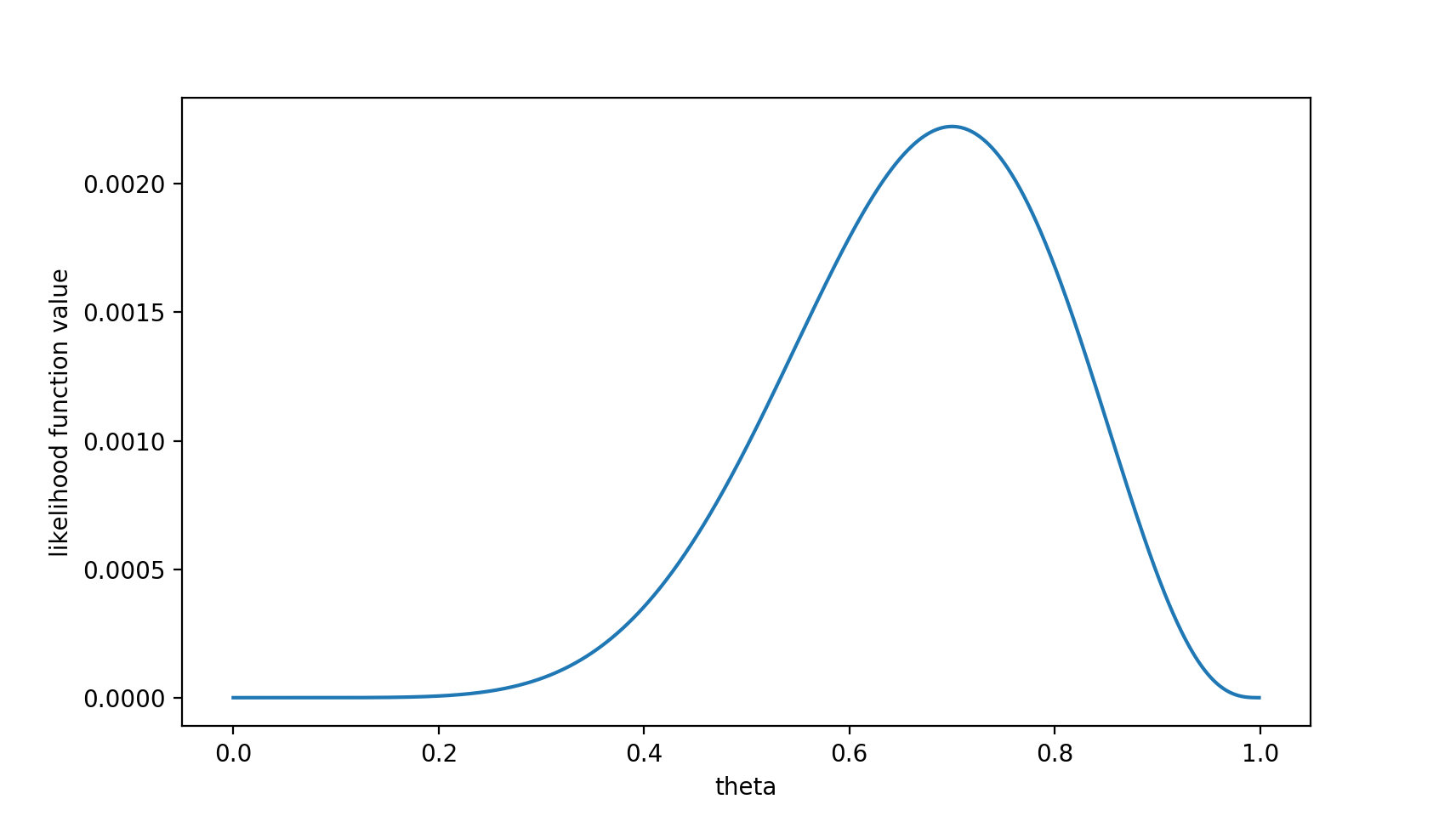可以看出，在

θ

=

0.7

\theta = 0.7

时，似然函数取得最大值。
这样，我们已经完成了对

θ

\theta

的最大似然估计。即，抛10次硬币，发现7次硬币正面向上，最大似然估计认为正面向上的概率是0.7。（ummm…这非常直观合理，对吧？）
且慢，一些人可能会说，硬币一般都是均匀的啊！ 就算你做实验发现结果是“反正正正正反正正正反”，我也不信

θ

=

0.7

\theta = 0.7

。
这里就包含了贝叶斯学派的思想了——要考虑先验概率。 为此，引入了最大后验概率估计。
最大后验概率估计
最大似然估计是求参数

θ

\theta

, 使似然函数$P(x_0 | \theta) 最 大 。 最 大 后 验 概 率 估 计 则 是 想 求 最大。最大后验概率估计则是想求 \theta 使 使 P(x_0 | \theta) P(\theta) 最 大 。 求 得 的 最大。求得的 \theta 不 单 单 让 似 然 函 数 大 ， 不单单让似然函数大， \theta$自己出现的先验概率也得大。 （这有点像正则化里加惩罚项的思想，不过正则化里是利用加法，而MAP里是利用乘法）
MAP其实是在最大化

P

(

θ

∣

x

0

)

=

P

(

x

0

∣

θ

)

P

(

θ

)

P

(

x

0

)

P(\theta|x_0) = \frac{P(x_0|\theta)P(\theta)}{P(x_0)}

，不过因为

x

0

x_0

是确定的（即投出的“反正正正正反正正正反”），

P

(

x

0

)

P(x_0)

是一个已知值，所以去掉了分母

P

(

x

0

)

P(x_0)

（假设“投10次硬币”是一次实验，实验做了1000次，“反正正正正反正正正反”出现了n次，则

P

(

x

0

)

=

n

/

1000

P(x_0) = n/1000

。总之，这是一个可以由数据集得到的值）。最大化

P

(

θ

∣

x

0

)

P(\theta | x_0)

的意义也很明确，

x

0

x_0

已经出现了，要求

θ

\theta

取什么值使

P

(

θ

∣

x

0

)

P(\theta | x_0)

最大。顺带一提，

P

(

θ

∣

x

0

)

P(\theta | x_0)

即后验概率，这就是“最大后验概率估计”名字的由来。
对于投硬币的例子来看，我们认为（”先验地知道“）

θ

\theta

取0.5的概率很大，取其他值的概率小一些。我们用一个高斯分布来具体描述我们掌握的这个先验知识，例如假设

P

(

θ

)

P(\theta)

为均值0.5，方差0.1的高斯函数，如下图：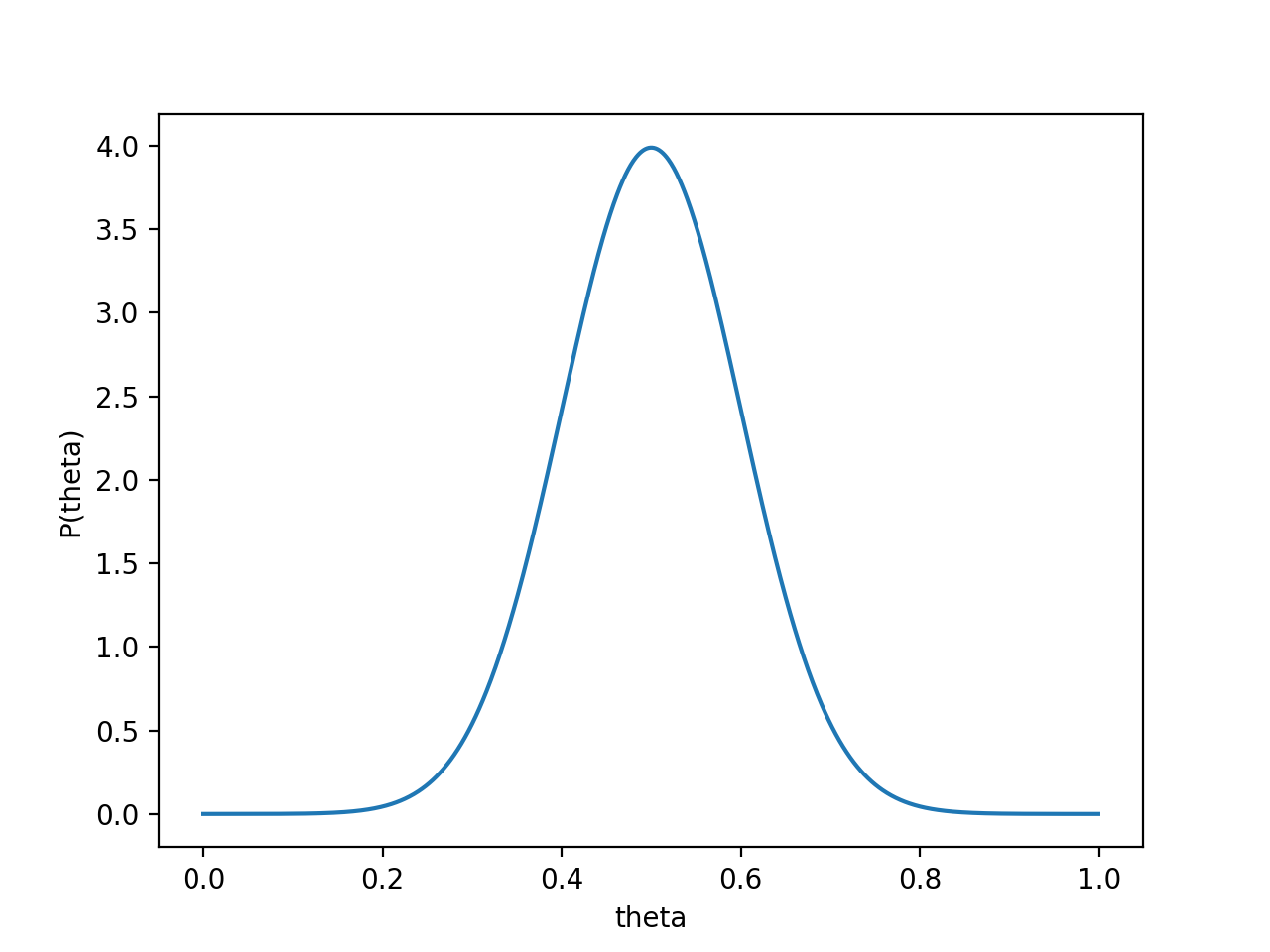则

P

(

x

0

∣

θ

)

P

(

θ

)

P(x_0 | \theta) P(\theta)

的函数图像为：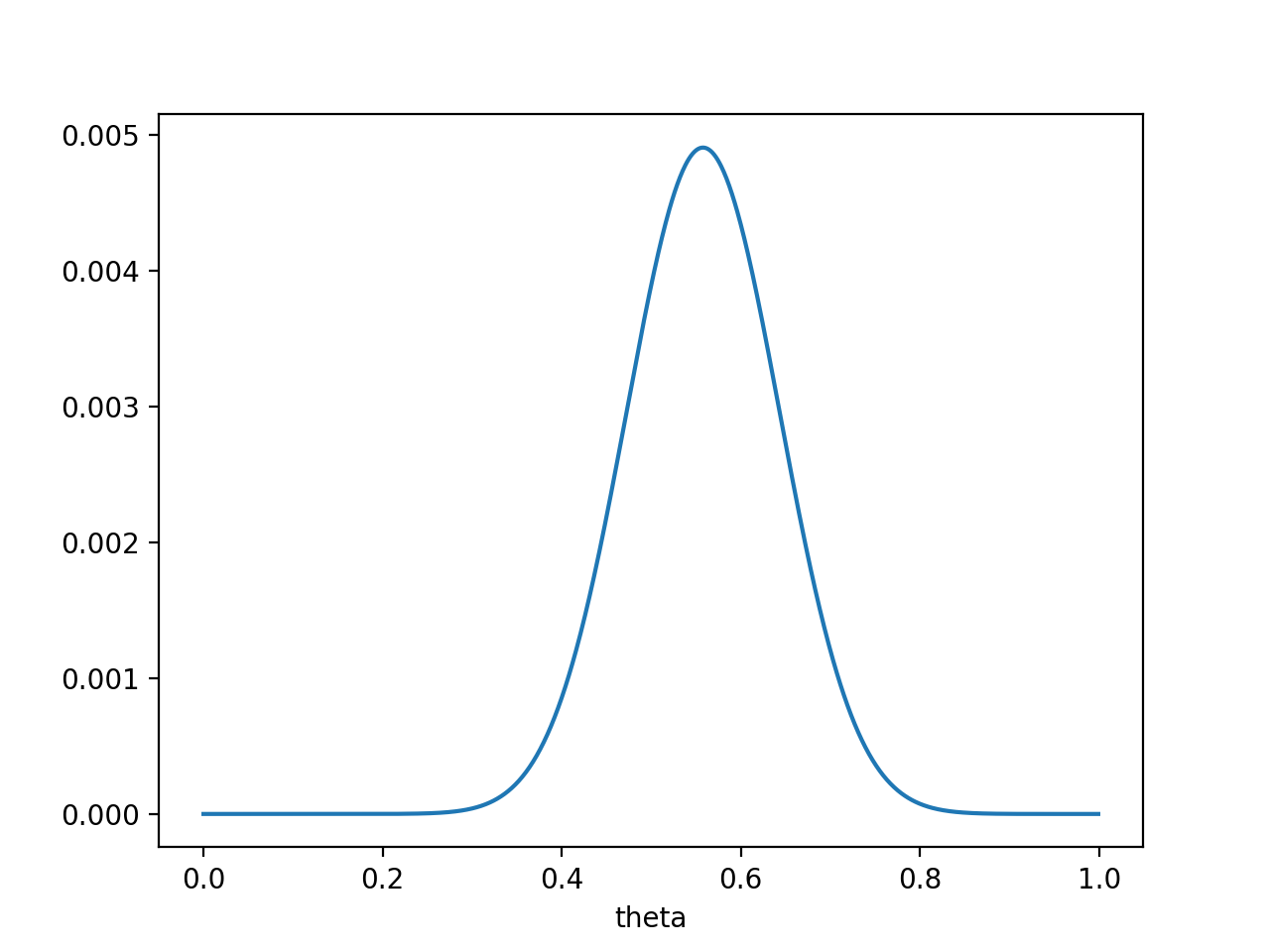注意，此时函数取最大值时，

θ

\theta

取值已向左偏移，不再是0.7。实际上，在

θ

=

0.558

\theta = 0.558

时函数取得了最大值。即，用最大后验概率估计，得到

θ

=

0.558

\theta = 0.558

最后，那要怎样才能说服一个贝叶斯派相信

θ

=

0.7

\theta = 0.7

呢？你得多做点实验。。
如果做了1000次实验，其中700次都是正面向上，这时似然函数为: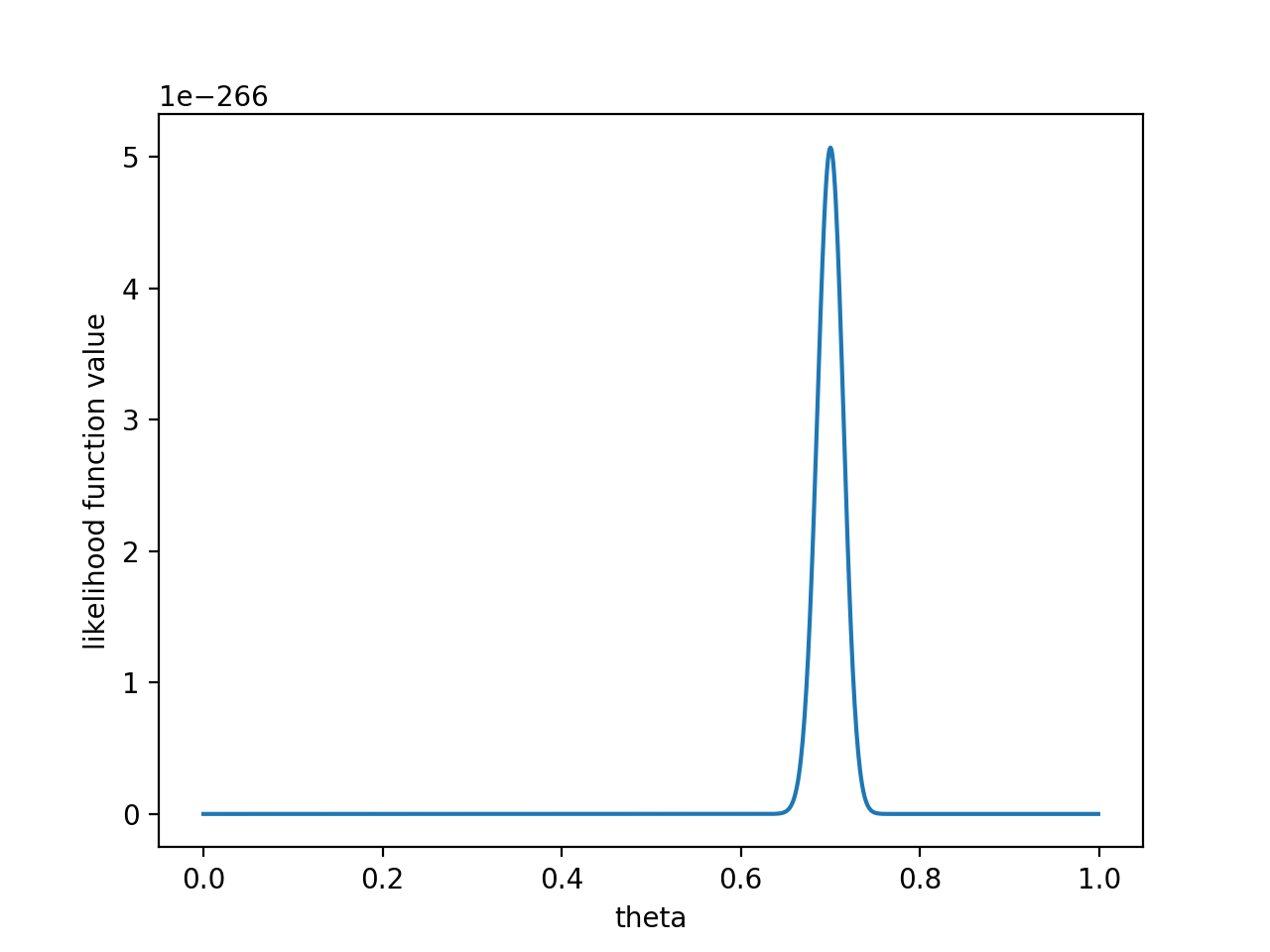如果仍然假设

P

(

θ

)

P(\theta)

为均值0.5，方差0.1的高斯函数，

P

(

x

0

∣

θ

)

P

(

θ

)

P(x_0 | \theta) P(\theta)

的函数图像为：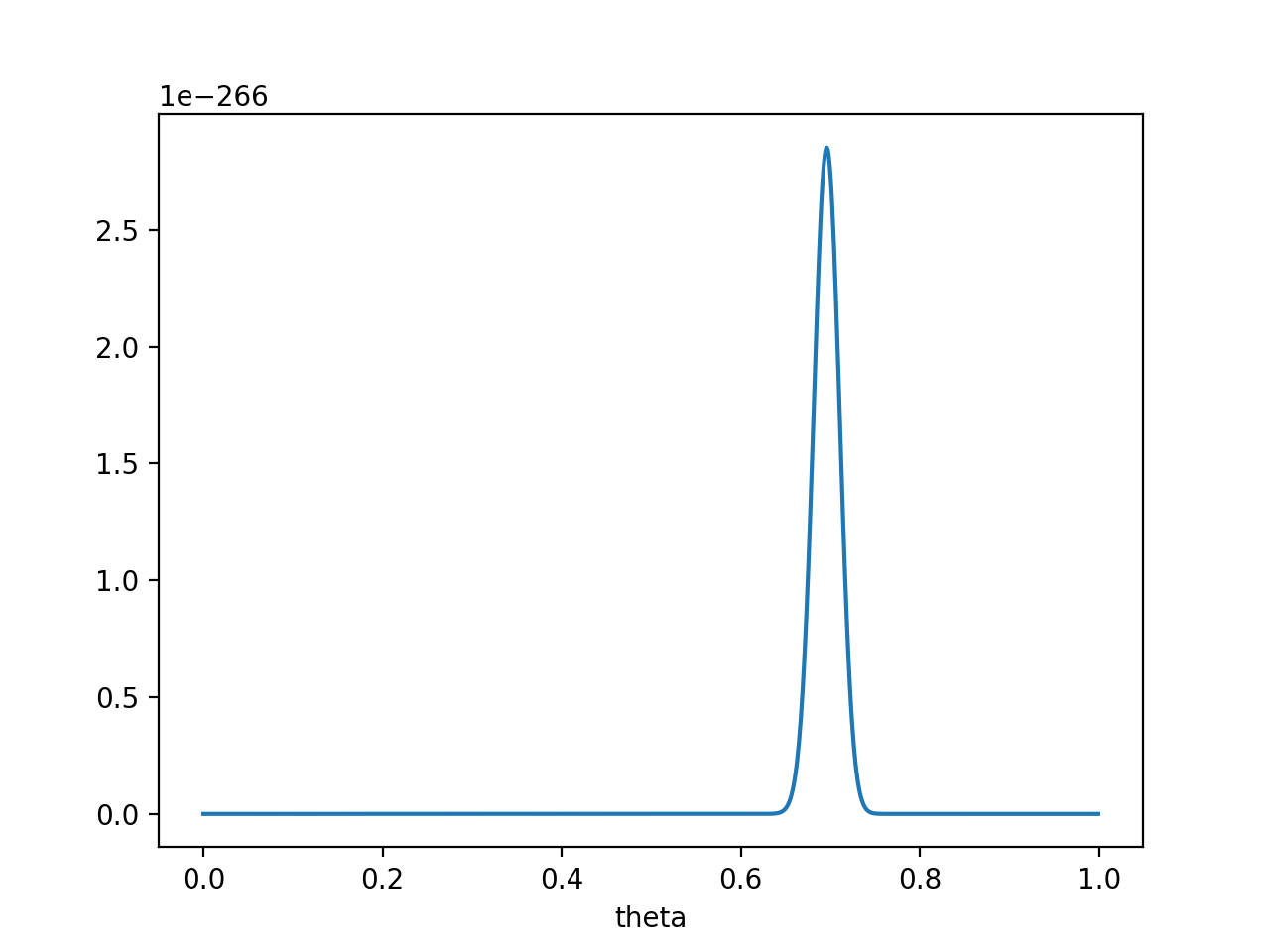在

θ

=

0.696

\theta = 0.696

处，

P

(

x

0

∣

θ

)

P

(

θ

)

P(x_0 | \theta) P(\theta)

取得最大值。
这样，就算一个考虑了先验概率的贝叶斯派，也不得不承认得把

θ

\theta

估计在0.7附近了。
PS. 要是遇上了顽固的贝叶斯派，认为

P

(

θ

=

0.5

)

=

1

P(\theta = 0.5) = 1

，那就没得玩了。。 无论怎么做实验，使用MAP估计出来都是

θ

=

0.5

\theta = 0.5

。这也说明，一个合理的先验概率假设是很重要的。（通常，先验概率能从数据中直接分析得到）
最大似然估计和最大后验概率估计的区别
相信读完上文，MLE和MAP的区别应该是很清楚的了。MAP就是多个作为因子的先验概率

P

(

θ

)

P(\theta)

。或者，也可以反过来，认为MLE是把先验概率

P

(

θ

)

P(\theta)

认为等于1，即认为

θ

\theta

是均匀分布。

如果有说错的或者没说清楚的地方，欢迎留言指教！如果您更好的见解，也欢迎留言交流！ 谢谢阅读！ 作者: nebulaf91
展开全文模式识别 机器学习 贝叶斯
• L1、L2 正则项详解 （解空间、先验分布） 引入 直观看 解空间 先验分布 最大似然估计 最大后验估计


L1、L2 正则项详解（解空间、先验分布）
引入直观看解空间先验分布最大似然估计最大后验估计

引入 在线性回归或其他模型中，我们经常使用正则项，例如：对普通线性回归添加 L1 正则项后就变为 Lasso 回归，对普通线性回归添加 L2 正则项后就变为 Ridge 回归。对于正则项，我们都知道 L2 正则项具有缩放型效应，可以让模型参数更加平滑；L1 正则项具有截断型效应，使得模型参数更加稀疏。那么本文就对这现象的背后原理进行讲解。  直观看 首先对平滑与稀疏建立一个直观的概念，稀疏意味着使模型参数等于 0，从而在模型内部嵌入式地进行特征选择,；而平滑则会尽量使参数小，但不等于 0，从而嵌入式地对特征进行缩小。 举一个不严谨但直观简单的例子，方便理解：设

f

(

x

;

w

)

f(x;w)

为经验损失，

L

L

代表正则项，

l

(

x

;

w

)

=

f

(

x

;

w

)

+

L

l(x;w)=f(x;w)+L

为结构损失。假设此时使用梯度下降更新参数，

μ

μ

为学习率，则梯度为

μ

∂

f

(

w

)

∂

w

i

−

μ

∗

∂

L

∂

w

i

μ\frac{\partial f(w)}{\partial w_i}-μ*\frac{\partial L}{\partial w_i}

，对于不同的正则项

μ

∂

f

(

w

)

∂

w

i

μ\frac{\partial f(w)}{\partial w_i}

是相同的，我们暂时忽略，所以：
使用 L1 正则项，梯度为：

μ

∂

∑

i

=

1

n

∣

w

i

∣

∂

w

i

=

μ

∗

1

=

μ

μ\frac{\partial \sum_{i=1}^{n}|w_i|}{\partial w_i}=μ*1=μ

， 梯度更新参数的表达式为：

w

i

:

=

w

i

−

μ

∗

1

w_i:=w_i-μ*1

，假设

μ

=

0.5

μ=0.5

，则

w

i

:

=

w

i

−

0.5

w_i:=w_i-0.5

，所以 L1 意味着在更新参数时相当于一直减去一个定值，那么终会有一时刻将

w

i

w_i

减为 0，那么此时参数为 0，所以稀疏。使用 L2 正则项，梯度为：

1

2

μ

∂

∑

i

=

1

n

(

w

i

)

2

∂

w

i

=

μ

w

i

\frac{1}{2}μ\frac{\partial \sum_{i=1}^{n}(w_i)^2}{\partial w_i}=μw_i

， 梯度更新参数的表达式为：

w

i

:

=

w

i

−

μ

w

i

w_i:=w_i-μw_i

，假设

μ

=

0.5

μ=0.5

，则

w

i

:

=

w

i

−

1

2

w

i

:

=

1

2

w

i

w_i:=w_i-\frac{1}{2}w_i:=\frac{1}{2}w_i

，相当于每次的更新都是折半，则更新的图像为

log

⁡

2

w

i

\log_2w_i

，所以只会趋近于 0，不会等于 0，趋于平滑。 通过上面不正规的例子，我们对稀疏性与平滑型有了直观的理解，下面就开始正式的通过公式证明。  解空间 在优化过程中，我们希望在优化经验损失

∑

i

=

1

n

(

y

i

−

w

T

x

i

)

2

\sum_{i=1}^{n}(y_i-w^Tx_i)^2

的同时，还希望对模型的复杂度进行限制，约束参数的取值空间，从而达到防止过拟合的效果，那么我们可以为优化问题加上一个约束，即参数

w

w

的范数不能大于 C： 对于 Lβ 范数：

{

m

i

n

∑

i

=

1

n

(

y

i

−

w

T

x

i

)

2

s

.

t

.

∣

∣

w

∣

∣

β

−

C

≤

0

，

β

=

1

,

2

\begin{cases} min\sum_{i=1}^{n}(y_i-w^Tx_i)^2\\ s.t. ||w||_β-C \le 0，β=1,2\\ \end{cases}

为了求解带约束条件的凸优化问题，写出拉格朗日函数：

L

(

x

,

w

,

λ

)

=

∑

i

=

1

n

(

y

i

−

w

T

x

i

)

2

+

λ

(

∣

∣

w

∣

∣

β

−

C

)

L(x,w,λ)=\sum_{i=1}^{n}(y_i-w^Tx_i)^2+λ(||w||_β-C)

假设此时

w

∗

,

λ

∗

w^*,λ^*

为原始问题与对偶问题的最优解，则根据

K

K

T

KKT

条件，应分别满足：

{

∇

w

L

(

x

∗

;

w

∗

;

λ

∗

)

=

0

∇

λ

L

(

x

∗

;

w

∗

;

λ

∗

)

=

0

λ

(

∣

∣

w

∣

∣

β

−

C

)

=

0

λ

∗

≥

0

∣

∣

w

∣

∣

β

−

C

≤

0

⟹

其

中

{

∇

w

[

∑

i

=

1

n

(

y

i

−

w

∗

T

x

i

∗

)

2

+

λ

∗

(

∣

∣

w

∗

∣

∣

β

−

C

)

]

=

0

λ

∗

≥

0

\begin{cases} \nabla_{w}L(x^*;w^*;λ^*)=0\\ \nabla_{λ}L(x^*;w^*;λ^*)=0\\ λ(||w||_β-C)=0\\ λ^*\ge0\\ ||w||_β-C\le0 \end{cases}\mathop{}_{\Longrightarrow}^{其中}\begin{cases} \nabla_{w}[\sum_{i=1}^{n}(y_i-{w^*}^Tx_i^*)^2+λ^*(||w^*||_β-C)]=0\\ λ^*\ge0\\ \end{cases}

回头看，

∇

w

[

∑

i

=

1

n

(

y

i

−

w

∗

T

x

i

∗

)

2

+

λ

∗

(

∣

∣

w

∗

∣

∣

β

−

C

)

]

=

0

\nabla_{w}[\sum_{i=1}^{n}(y_i-{w^*}^Tx_i^*)^2+λ^*(||w^*||_β-C)]=0

表示的就是：

w

∗

,

λ

∗

w^*,λ^*

为带有正则项的优化问题的最优解的条件，且

λ

∗

λ^*

为正则项前的正则参数。与我们通常使用的结构损失正好对应！ 对于正则项前的系数

λ

λ

，用来控制经验损失与正则项的相对重要性，

λ

λ

值越大，表明正则项越严格，更倾向于获得稀疏模型。 由以上我们可以得出：加入正则项就相当于对经验损失加上约束条件，从而变成约束优化问题。对应于解空间来讲，有下图：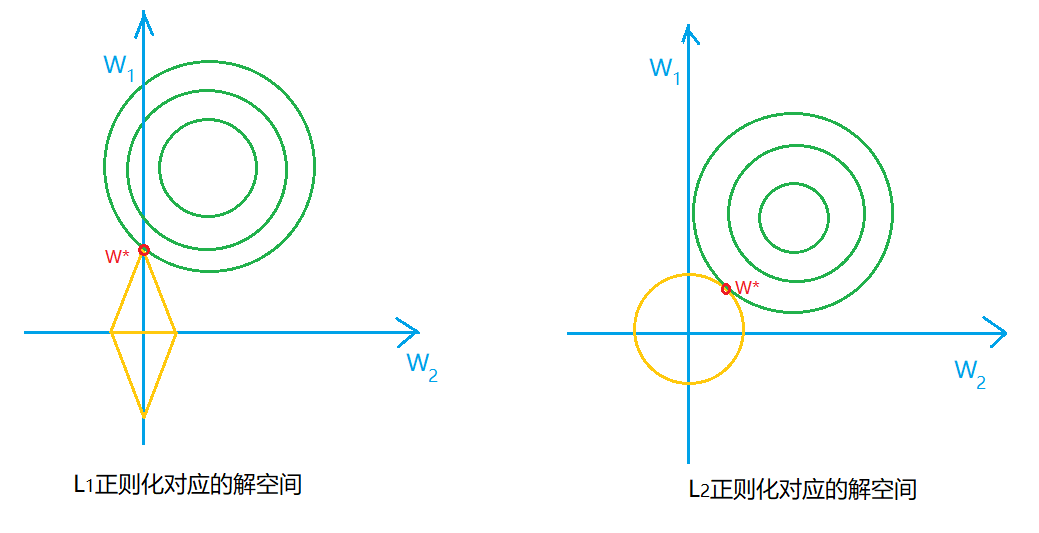对于解空间我们可以想象到 2 维的情景，假设在

w

1

,

w

2

w_1,w_2

的解空间中，菱形与圆形分别对应 L1 与 L2 中

w

1

,

w

2

w_1,w_2

取值范围，

w

∗

w^*

为

w

w

的最优解（L1 正则是绝对值之和，可以认为是一次函数类似

a

w

1

+

b

w

2

=

0

aw_1+bw_2=0

围城的图形，所以是菱形；L1 正则是平方之和，可以认为是二次函数类似

(

w

1

)

2

+

(

w

2

)

2

=

0

(w_1)^2+(w_2)^2=0

围城的图形，所以是圆形）。 如果原问题目标函数的最优解不是恰好落在解空间内，那么约束条件下的最优解必然在解空间与约束空间的交点处。L1 “棱角分明”的约束空间更容易与解空间在角点碰撞，且 L1 中两个参数值倾向于一个较大另一个为 0，L2 中两个参数倾向于均为非零的较小数（使用 L2 正则项时，只有当解空间的中心与 L2 约束空间的中心垂直或平行时才能得到一个参数为 0，另一个参数较大，而 L1 的约束空间则不然）。所以就有 L1 稀疏，L2 平滑的效果。  先验分布 如果在概率估计的范畴，则可以通过先验分布解释 L1 与 L2 的稀疏性与平滑性。 在从先验分布角度介绍 L1，L2 之前，我们先了解一下最大似然估计与最大后验估计（参考链接）。
最大似然估计 对于函数

P

(

x

∣

θ

)

P(x|θ)

，有两个输入，

x

x

表示已知数据，

θ

θ

表示条件参数，那么这个条件概率可以从两个方面理解：

∙

\bullet

如果

θ

θ

已知，

x

x

未知，这个函数称为概率函数，用于计算对于不同

x

x

发生的概率；

∙

\bullet

如果

x

x

已知，

θ

θ

未知，这个函数称为似然函数，用来描述，当样本数据发生的经验概率（可从数据中统计）是已知，计算使它产生这样经验概率的参数是多少（似然，可以认为是接近真实，近似它自然拥有的参数）。 最大似然估计的计算过程：

例：此时抛硬币10次，其中正面 7 次，反面 3 次，设 硬币正面出现的概率为

θ

θ

， 请估计硬币正面出现的概率。
① 写出 似然函数：

f

(

x

)

=

θ

7

(

1

−

θ

)

3

f(x)=θ^7(1-θ)^3

② 转换成 对数似然函数：

ln

⁡

f

(

x

)

=

ln

⁡

(

θ

7

(

1

−

θ

)

3

)

=

7

ln

⁡

θ

+

3

ln

⁡

(

1

−

θ

)

\ln f(x)=\ln (θ^7(1-θ)^3)=7\ln θ + 3\ln(1-θ)

③ 对

θ

θ

求导，并令导数等于 0：

7

θ

−

3

1

−

θ

=

0

\frac{7}{θ}-\frac{3}{1-θ}=0

，解得

θ

=

0.7

θ=0.7

（④ 对于更加复杂的函数形式可使用梯度下降）  最大后验估计 最大后验估计运用贝叶斯公式：

P

(

w

∣

X

)

=

P

(

X

∣

w

)

P

(

w

)

P

(

X

)

P(w|X)=\frac{P(X|w)P(w)}{P(X)}

，是在最大似然估计的基础上加入了先验概率。 其中

X

X

作为已知的训练数据出现，最大似然估计的目的是最大化

P

(

w

∣

X

)

P(w|X)

，即在已知数据的基础上既最大化似然函数

P

(

X

∣

w

)

P(X|w)

，又要最大化

P

(

w

)

P(w)

。意味着要考虑先验概率

P

(

w

)

P(w)

。与之前文章讲的贝叶斯公式（机器学习 - 朴素贝叶斯（上）- 概率论基础）可视为一样的：我们先对参数

w

w

计算先验概率，而后通过数据计算条件概率

P

(

X

∣

w

)

P(X|w)

对先验概率进行修正。  现在我们可以根据最大后验估计对 L1 与 L2 的稀疏与平滑进行解释了。 对于机器学习模型，我们都是已知数据对参数进行学习，相当于对

w

w

进行估计，此过程可用最大后验估计表示，其中

X

,

Y

X,Y

为数据及其标签：

P

(

w

∣

X

,

Y

)

=

P

(

X

,

Y

∣

w

)

P

(

w

)

P

(

X

,

Y

)

P(w|X,Y)=\frac{P(X,Y|w)P(w)}{P(X,Y)}

根据最大后验估计，我们的目标是最大化

P

(

X

,

Y

∣

w

)

P(X,Y|w)

以及

P

(

w

)

P(w)

，而对于同一个任务，所使用的数据是不变的，所以

P

(

X

,

Y

)

P(X,Y)

可以视为常数忽略，此时最大后验估计可表示为：

P

(

w

∣

X

,

Y

)

=

P

(

X

,

Y

∣

w

)

P

(

w

)

P(w|X,Y)=P(X,Y|w)P(w)

同样取对数，得对数后验函数：

f

(

x

)

=

log

⁡

(

P

(

X

,

Y

∣

w

)

P

(

w

)

)

=

log

⁡

P

(

X

,

Y

∣

w

)

+

log

⁡

P

(

w

)

f(x)=\log (P(X,Y|w)P(w))=\log P(X,Y|w)+\log P(w)

从中可以发现：对数后验函数是在对数似然函数的基础上增加了

log

⁡

P

(

w

)

\log P(w)

，其中

P

(

w

)

P(w)

的意义是对参数

w

w

的概率分布做出的先验假设，在收集到训练数据

X

,

Y

X,Y

后，则可根据

w

w

在

X

,

Y

X,Y

下的条件概率对

w

w

进行修正，从而做出对

w

w

进行更好地估计。

∙

\bullet

若假设

w

j

w_j

的先验分布为 0均值 的高斯分布，即：

w

j

∼

N

(

0

,

σ

2

)

w_j\sim N(0,σ^2)

log

⁡

P

(

w

)

=

log

⁡

∏

j

P

(

w

j

)

=

log

⁡

∏

j

[

−

1

2

π

σ

2

e

−

(

w

j

)

2

2

σ

2

]

=

−

1

2

σ

2

∑

j

w

j

2

+

C

′

\log P(w)=\log \prod_{j} P(w_j)=\log \prod_{j}[-\frac{1}{\sqrt{2π}σ^2}e^{-\frac{(w_j)^2}{2σ^2}}]=-\frac{1}{2σ^2}\sum_{j}w_j^2+C&#x27;

很巧，在高斯分布下，

log

⁡

P

(

w

)

\log P(w)

具有

∑

j

w

j

2

\sum_{j}w_j^2

项 ，与 L2 正则项是效果等价的。 所以使用 L2 正则项相当于对模型参数

w

w

引入了高斯先验。

∙

\bullet

若假设

w

j

w_j

服从 均值为0，参数为

a

a

的拉普拉斯分布，即：

P

(

w

j

)

=

1

2

a

e

−

∣

w

j

∣

a

P(w_j)=\frac{1}{\sqrt{2a}}e^{\frac{-|w_j|}{a}}

log

⁡

P

(

w

)

=

log

⁡

∏

j

P

(

w

j

)

=

log

⁡

∏

j

1

2

a

e

−

∣

w

j

∣

a

=

−

1

a

∑

j

∣

w

j

∣

+

C

′

\log P(w)=\log \prod_{j} P(w_j)=\log \prod_{j}\frac{1}{\sqrt{2a}}e^{\frac{-|w_j|}{a}}=-\frac{1}{a}\sum_{j}|w_j|+C&#x27;

很巧，在拉普拉斯分布下，

log

⁡

P

(

w

)

\log P(w)

具有

∑

j

∣

w

j

∣

\sum_{j}|w_j|

项 ，与 L1 正则项是效果等价的。 所以使用 L1 正则项相当于对模型参数

w

w

引入了拉普拉斯先验。 但是高斯先验为什么会使参数更平滑而拉普拉斯为什么会使参数更稀疏呢？ 首先来看阿门两个分布函数的图像：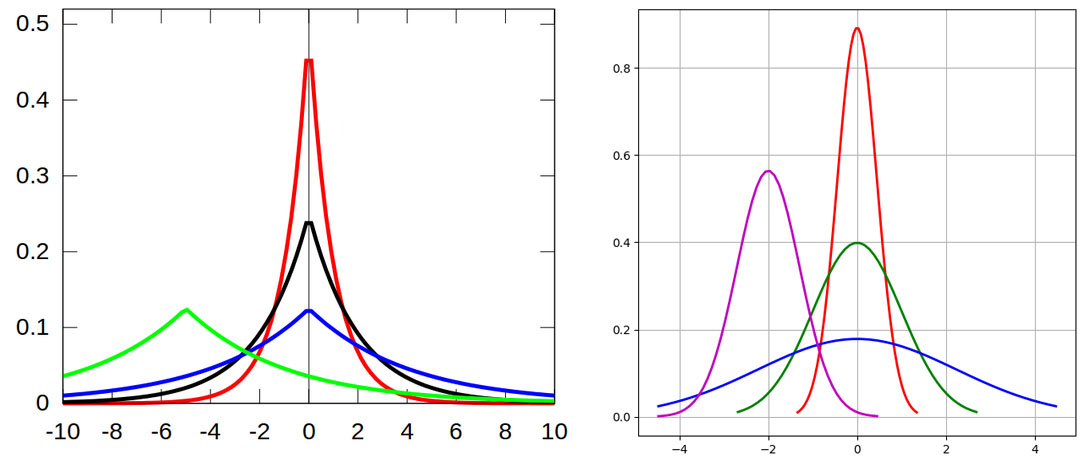（左图为拉普拉斯分布，右图为高斯分布，图片来自网络。） 对于拉普拉斯分布，大部分概率分布都聚集在 0 附近，而对于值较大的概率较小，所以获得

w

=

0

w=0

的概率更高，从而更倾向于稀疏；而对于高斯分布，其中取值较大的概率的分布相比拉普拉斯分布更大，而在 0 附近的概率分布较小，所以对于

w

=

0

w=0

的概率更小，所以更倾向于平滑。 
展开全文L1、L2正则化 解空间 先验假设
•机器学习
• 前言：机器学习系列文章常含有大量公式，若需获取本文全部的手书版原稿资料，扫码关注公众号【AI机器学习与知识图谱】，回复: 高斯分布第一讲 即可获取。 原创不易，转载请告知并注明出处！扫码关注公众号，定期发布...机器学习 算法
• 前言 参数估计是机器学习里面的一个重要主题，而极大似然估计是最传统、使用最广泛的估计方法之一。...这时候我们就需要用极大似然估计来求解这个分布的参数。换句话说，极大似然估计提供了一种给定观察数据来评...
• ## 多元正态分布的极大似然估计

万次阅读 多人点赞 2018-06-20 13:53:12
多元正态分布极大似然估计 1. 一元正态分布的密度函数 一元正态分布的密度函数表示为： f(x)=1(2π)−−−−√σe−(x−μ)22σ2f(x)=1(2π)σe−(x−μ)22σ2f(x) = \frac{1}{\sqrt {(2 \pi)} \sigma} e^{...
• 1、极大似然估计是一种目前仍然得到广泛应用的方法。它是建立在极大似然原理的基础上的一个统计方法，极大似然原理的直观想法是：一个随机试验如有若干个可能的结果A，B，C，…。若在一次试验中，结果A出现，则一般...
• 极大似然估计法是求估计的另一种方法。它最早由高斯提出。后来为费歇在1912年的文章中重新提出，并且证明了这个方法的一些性质。极大似然估计这一名称也是费歇给的。这是一种上前仍然得到广泛应用的方法。它是建立在...
• 回归问题普遍讨论的是多元线性回归，考虑多个特征可以得到更精确的模型，这其中涉及中心极限定理，正态分布，概率密度函数和最大似然估计。 (一)背景——多元线性回归 1. 本质上就是算法（公式）变换为了多元一次...机器学习 多元线性回归
• 极大似然估计**是神经网络和很多复杂模型得以优化求解的理论基础, 我们今天来学习并试着深入理解极大似然估计的原理和推导, 最后我们对极大似然估计进行3D可视化, 建立一种直观的认识. 要理解极大似然估计是什么, ...似然估计 似然函数
• 1.极大似然估计（也称最大似然估计） 模型和观察数据X已知，模型参数未知。假设所有采样都是独立同分布的，得到让观察样本出现的概率最大的参数。 的最大似然估计： 求最大似然函数估计值的一般步骤： （1）...
• 伯努利分布（Bernoulli distribution，又名两点分布或者0-1分布，是一个离散型概率分布，为纪念瑞士科学家雅各布·伯努利而命名。)若伯努利试验成功，则伯努利随机变量取值为0。记其成功概率为 p(0≤p≤1){\...贝叶斯估计 参数估计
• ## 最大似然估计详解

万次阅读 多人点赞 2017-05-18 15:07:12
最大似然估计是建立在最大似然...这里用到了”概率最大的事件最可能出现”的直观想法，然后对Ak出现的概率公式求极大值，这样便可解未知参数。下面用一个例子说明最大似然估计的思想方法。  假设一个
• 高斯分布 就是我们常说的正态分布，也叫常态分布，名字有很多~~后面统一叫高斯分布。 图形非常的常见~ 最简单的，人类的身高分布，学习成绩这种，基本都服从于高斯分布。 一维高斯分布： ...极大似然估计 第一次深度学习 人工智能 数学
• 极大似然估计个人理解 极大似然估计属于频率派统计。 极大似然估计理解: 对给定的一组样本，对他的分布进行估计。 拿正态分布来说：上帝（知道正态分布里真正的的μ和θ比如μ=0，θ=0.4）从这个分布里拿出N个样本...机器学习基础知识
• 极大似然估计法是求估计的另一种方法。它最早由高斯提出。后来为费歇在1912年的文章中重新提出，并且证明了这个方法的一些性质。极大似然估计这一名称也是费歇给的。这是一种上前仍然得到广泛应用的方法。它是建立在...
• 1、最大似然估计 似然的概念与概率类似，但是又很不相同。假如随机变量X服从某种分布（比如高斯分布），概率是指在给定参数（均值，方差）的条件下，X=x的可能性；而似然则指X=x的条件下，某一组参数反映了X=x的真实...EM算法 高斯混合模型
• ## 极大似然估计与贝叶斯估计

万次阅读 多人点赞 2016-10-11 14:04:58
极大似然估计与贝叶斯估计是统计中种对模型的参数确定的方法，种参数估计方法使用不同的思想。前者来自于频率派，认为参数是固定的，我们要做的事情就是根据已经掌握的数据来估计这个参数；而后者属于贝叶斯派，...机器学习 贝叶斯估计 参数估计
• 2极大似然估计 2.1什么是极大似然估计 2.2极大似然原理及数学表示 2.3极大似然估计法(Maximum Likelihood Estimation,MLE) 2.3.1总体X为离散型 2.3.2总体X为连续型 2.4极大似然估计法求估计值 3极大似然估计......